• 零售商以 $p$ 元/件的采购价 (purchase price) 从供应商处采购某种商品, 以 $r$ 元/件的零售价 (retail price) 售卖给消费者, 其中 $r>p$.
• 零售商将商品存放在仓库中, 为此需要承担 $h$ 元/(件·天) 的持有成本 (holding cost).
• 考虑到运费、人力等因素, 每次从供应商处补货, 零售商都需承担 $K$ 元的固定成本 (fixed setup cost).
• 消费者不接受延迟发货, 这意味着当库存量无法满足消费者的需求时, 零售商就要承担缺货损失 (shortage cost).
• 消费者对商品的需求量是随机的, 但未来任意一段时间的需求量所服从的概率分布是已知的.
• 零售商从供应商处订货的提前期 (lead time) $L$ 天, 最小起订量为 $moq$ 件.
• 零售商采用 (R, Q) 订货策略, 即当库存量下降到再订货点 (Reorder Point) $R$ 时, 就触发补货, 订货量 (Order Quantity) 为 $Q$. 此外, 由于操作上的原因, 两次订货之间的时间间隔不能少于 $\tau$ 天. 零售商希望尽可能维持 $\alpha$ 的服务水平 (service level) , 同时尽可能降低成本, 需要由算法来决策 $R$$Q$.

## 求解

### 确定再订货点

\begin{aligned}f_{\tau_1, \tau_2}(x) &= Prob(D_{\tau_1, \tau_2}= x) \\F_{\tau_1, \tau_2}(x) &= Prob(D_{\tau_1, \tau_2}\leq x)\end{aligned}

$t_0$ 表示当前时刻, $s_0$ 表示当前的库存. 用 $(t_1, s_1), (t_2, s_2), \cdots, (t_n, s_n)$ 表示在途库存, 即 $t_i$ 时刻将会入库 $s_i$ 单位货物. 这里 $t_0.

\begin{aligned}\tilde\alpha &= Prob(D_{t_0, t_0+L}\leq s_0) \\&= F_{t_0, t_0+L}(s_0)\end{aligned}

\begin{aligned}\tilde\alpha &= Prob((D_{t_0, t_1}\leq s_0)\cap (D_{t_0, t_0+L}\leq s_0+s_1)) \\&= Prob((\bigcup_{x=0}^{s_0}D_{t_0, t_1}=x)\cap (D_{t_0, t_0+L}\leq s_0+s_1)) \\&= Prob(\bigcup_{x=0}^{s_0}((D_{t_0, t_1}=x)\cap (D_{t_0, t_0+L}\leq s_0+s_1))) \\&= Prob(\bigcup_{x=0}^{s_0}((D_{t_0, t_1}=x)\cap (D_{t_1, t_0+L}\leq s_0+s_1-x))) \\&= \sum_{x=0}^{s_0}Prob((D_{t_0, t_1}=x)\cap (D_{t_1, t_0+L}\leq s_0+s_1-x)) \\&= \sum_{x=0}^{s_0}Prob(D_{t_0, t_1} = x)\cdot Prob(D_{t_1, t_0+L}\leq s_0+s_1-x) \\&=\sum_{x=0}^{s_0}f_{t_0, t_1}(x)\cdot F_{t_1, t_0+L}(s_0+s_1-x)\end{aligned}

“The calculation … is in principle very simple; it is easier to do it oneself than to follow an account of it. Hence we shall give only the result of this calculation.”

$\tilde\alpha = \sum_{x_0=0}^{s_0}f_{t_0, t_1}(x_0)\sum_{x_1=0}^{s_0+s_1-x_0}f_{t_1, t_2}(x_1)\cdots\sum_{x_{n-1}=0}^{\sum_{i=0}^{n-1}s_i-\sum_{i=0}^{n-2}x_i}f_{t_{n-1}, t_n}(x_{n-1})F_{t_n, t_0+L}(\sum_{i=0}^{n}s_i-\sum_{i=0}^{n-1}x_i)$

### 确定订货量

$t_0$ 天订货 $Q$ 件, 第 $t_0+L$ 天到货, 假设这批货到第 $t_0+L+t$ 天消耗完(库存先进先出), 则

• 采购成本为 $p\cdot Q + K$, 平摊到每天为 $\dfrac{p\cdot Q + K}{t+1}$.

• 持有成本从入库开始计算, 直至这批货消耗完为止, 平摊到每天约等于 $\dfrac{h\cdot Q}{2}$.

• 缺货损失从入库开始计算, 直至 $\tau$ 天后为止.

• $t<\tau$ 时, 缺货损失为单位利润 $r-p$ 乘以 $[t_0+L+t, t_0+L+\tau)$ 内的期望需求量 $\mathbb ED_{t_0+L+t, t_0+L+\tau}$.
• $t\geq\tau$ 时, 缺货损失为 $0$.

为了统一形式, 我们规定当 $\tau_2\leq \tau_1$ 时, $D_{\tau_1, \tau_2}\equiv 0$. 这样一来, 缺货损失为 $(r-p)\cdot\mathbb ED_{t_0+L+t, t_0+L+\tau}$, 平摊到每天为 $\dfrac{(r-p)\cdot\mathbb ED_{t_0+L+t, t_0+L+\tau}}{t+1}$.

$cost(Q) = \sum_{t=0}^{\infty}p(t)\left[\frac{p\cdot Q + K}{t+1}+ \frac{h\cdot Q}{2}+\frac{(r-p)\cdot\mathbb ED_{t_0+L+t, t_0+L+\tau}}{t+1}\right]$

\begin{aligned}p(t) &= Prob(D_{t_0, L}>s_0)\cdot Prob(D_{t_0+L, t_0+L+t}\leq Q< D_{t_0+L, t_0+L+t+1}) \\&+ \sum_{x=0}^{s_0}Prob(D_{t_0, L}=x)\cdot Prob(D_{t_0+L, t_0+L+t}\leq Q+s_0-x< D_{t_0+L, t_0+L+t+1})\\&=(1-F_{t_0, L}(s_0))\cdot (F_{t_0+L, t_0+L+t}(Q)-F_{t_0+L, t_0+L+t+1}(Q))\\&+\sum_{x=0}^{s_0}f_{t_0, L}(x)\cdot(F_{t_0+L, t_0+L+t}(Q+s_0-x)-F_{t_0+L, t_0+L+t+1}(Q+s_0-x))\\\end{aligned}

1. 提前期指从下单开始到入库为止, 所经历的时间. Lead time is the amount of time between the placement of and order to replenish inventory and the receipt of the goods into inventory. ↩︎

2. 服务水平指从下单开始到入库为止, 期间不发生缺货的概率. Service level is the probability that a stockout will not occur between the time an order is placed and the order quantity is received. ↩︎

]]>
<h2 id="问题"><a class="markdownIt-Anchor" href="#问题"></a> 问题</h2> <p>考虑一个商品补货问题:</p> <blockquote> <ul> <li>零售商以 <span class="katex"><span class="katex-mathml">$<semantics><mrow><mi>p</mi></mrow><annotation encoding="application/x-tex">p</annotation></semantics>$</span><span class="katex-html" aria-hidden="true"><span class="base"><span class="strut" style="height:0.625em;vertical-align:-0.19444em;"></span><span class="mord mathdefault">p</span></span></span></span> 元/件的采购价 (purchase price) 从供应商处采购某种商品, 以 <span class="katex"><span class="katex-mathml">$<semantics><mrow><mi>r</mi></mrow><annotation encoding="application/x-tex">r</annotation></semantics>$</span><span class="katex-html" aria-hidden="true"><span class="base"><span class="strut" style="height:0.43056em;vertical-align:0em;"></span><span class="mord mathdefault" style="margin-right:0.02778em;">r</span></span></span></span> 元/件的零售价 (retail price) 售卖给消费者, 其中 <span class="katex"><span class="katex-mathml">$<semantics><mrow><mi>r</mi><mo>&gt;</mo><mi>p</mi></mrow><annotation encoding="application/x-tex">r&gt;p</annotation></semantics>$</span><span class="katex-html" aria-hidden="true"><span class="base"><span class="strut" style="height:0.5782em;vertical-align:-0.0391em;"></span><span class="mord mathdefault" style="margin-right:0.02778em;">r</span><span class="mspace" style="margin-right:0.2777777777777778em;"></span><span class="mrel">&gt;</span><span class="mspace" style="margin-right:0.2777777777777778em;"></span></span><span class="base"><span class="strut" style="height:0.625em;vertical-align:-0.19444em;"></span><span class="mord mathdefault">p</span></span></span></span>.</li> <li>零售商将商品存放在仓库中, 为此需要承担 <span class="katex"><span class="katex-mathml">$<semantics><mrow><mi>h</mi></mrow><annotation encoding="application/x-tex">h</annotation></semantics>$</span><span class="katex-html" aria-hidden="true"><span class="base"><span class="strut" style="height:0.69444em;vertical-align:0em;"></span><span class="mord mathdefault">h</span></span></span></span> 元/(件·天) 的持有成本 (holding cost).</li> <li>考虑到运费、人力等因素, 每次从供应商处补货, 零售商都需承担 <span class="katex"><span class="katex-mathml">$<semantics><mrow><mi>K</mi></mrow><annotation encoding="application/x-tex">K</annotation></semantics>$</span><span class="katex-html" aria-hidden="true"><span class="base"><span class="strut" style="height:0.68333em;vertical-align:0em;"></span><span class="mord mathdefault" style="margin-right:0.07153em;">K</span></span></span></span> 元的固定成本 (fixed setup cost).</li> <li>消费者不接受延迟发货, 这意味着当库存量无法满足消费者的需求时, 零售商就要承担缺货损失 (shortage cost).</li> <li>消费者对商品的需求量是随机的, 但未来任意一段时间的需求量所服从的概率分布是已知的.</li> <li>零售商从供应商处订货的提前期 (lead time) <sup class="footnote-ref"><a href="#fn1" id="fnref1"></a></sup>为 <span class="katex"><span class="katex-mathml">$<semantics><mrow><mi>L</mi></mrow><annotation encoding="application/x-tex">L</annotation></semantics>$</span><span class="katex-html" aria-hidden="true"><span class="base"><span class="strut" style="height:0.68333em;vertical-align:0em;"></span><span class="mord mathdefault">L</span></span></span></span> 天, 最小起订量为 <span class="katex"><span class="katex-mathml">$<semantics><mrow><mi>m</mi><mi>o</mi><mi>q</mi></mrow><annotation encoding="application/x-tex">moq</annotation></semantics>$</span><span class="katex-html" aria-hidden="true"><span class="base"><span class="strut" style="height:0.625em;vertical-align:-0.19444em;"></span><span class="mord mathdefault">m</span><span class="mord mathdefault">o</span><span class="mord mathdefault" style="margin-right:0.03588em;">q</span></span></span></span> 件.</li> <li>零售商采用 (R, Q) 订货策略, 即当库存量下降到再订货点 (<strong>R</strong>eorder Point) <span class="katex"><span class="katex-mathml">$<semantics><mrow><mi>R</mi></mrow><annotation encoding="application/x-tex">R</annotation></semantics>$</span><span class="katex-html" aria-hidden="true"><span class="base"><span class="strut" style="height:0.68333em;vertical-align:0em;"></span><span class="mord mathdefault" style="margin-right:0.00773em;">R</span></span></span></span> 时, 就触发补货, 订货量 (Order <strong>Q</strong>uantity) 为 <span class="katex"><span class="katex-mathml">$<semantics><mrow><mi>Q</mi></mrow><annotation encoding="application/x-tex">Q</annotation></semantics>$</span><span class="katex-html" aria-hidden="true"><span class="base"><span class="strut" style="height:0.8777699999999999em;vertical-align:-0.19444em;"></span><span class="mord mathdefault">Q</span></span></span></span>. 此外, 由于操作上的原因, 两次订货之间的时间间隔不能少于 <span class="katex"><span class="katex-mathml">$<semantics><mrow><mi>τ</mi></mrow><annotation encoding="application/x-tex">\tau</annotation></semantics>$</span><span class="katex-html" aria-hidden="true"><span class="base"><span class="strut" style="height:0.43056em;vertical-align:0em;"></span><span class="mord mathdefault" style="margin-right:0.1132em;">τ</span></span></span></span> 天. 零售商希望尽可能维持 <span class="katex"><span class="katex-mathml">$<semantics><mrow><mi>α</mi></mrow><annotation encoding="application/x-tex">\alpha</annotation></semantics>$</span><span class="katex-html" aria-hidden="true"><span class="base"><span class="strut" style="height:0.43056em;vertical-align:0em;"></span><span class="mord mathdefault" style="margin-right:0.0037em;">α</span></span></span></span> 的服务水平 (service level) <sup class="footnote-ref"><a href="#fn2" id="fnref2"></a></sup>, 同时尽可能降低成本, 需要由算法来决策 <span class="katex"><span class="katex-mathml">$<semantics><mrow><mi>R</mi></mrow><annotation encoding="application/x-tex">R</annotation></semantics>$</span><span class="katex-html" aria-hidden="true"><span class="base"><span class="strut" style="height:0.68333em;vertical-align:0em;"></span><span class="mord mathdefault" style="margin-right:0.00773em;">R</span></span></span></span> 和 <span class="katex"><span class="katex-mathml">$<semantics><mrow><mi>Q</mi></mrow><annotation encoding="application/x-tex">Q</annotation></semantics>$</span><span class="katex-html" aria-hidden="true"><span class="base"><span class="strut" style="height:0.8777699999999999em;vertical-align:-0.19444em;"></span><span class="mord mathdefault">Q</span></span></span></span>.</li> </ul> </blockquote>## 决策模型

$(1+x)^k = \tbinom{k}{0}x^0 + \tbinom{k}{1}x^1 +\tbinom{k}{2}x^2 +\cdots+\tbinom{k}{k}x^k$

$x$ 很小时，忽略二阶小量，有

$(1+x)^k\approx1+kx$

$1-(1-p_i^{push})^k\approx 1- (1-kp_i^{push})=kp_i^{push}$

① 只给这部分用户发送短信，不发送 Push，则其被召回的概率是 $p_i^{sms}$
② 同时给这部分用户发送短信和 Push，则其被召回的概率是

$1-(1-p_i^{sms})(1-p_i^{push})^k\approx1-(1-p_i^{sms})(1-kp_i^{push})$

• 使用策略 ① 时，平均召回的用户数为

\begin{aligned}recall &= \sum_ip_i^{sms}X_i+\sum_ikp_i^{push}(1-X_i)\\&=\sum_i(p_i^{sms}-kp_i^{push})X_i+\sum_ikp_i^{push}\end{aligned}

$max \frac{\sum_i(p_i^{sms}-kp_i^{push})X_i+\sum_ikp_i^{push}}{\sum_iX_i}\\s.t. \quad\sum_iX_i\leq N$

• 使用策略 ② 时，平均召回的用户数为

\begin{aligned}recall &= \sum_i\left[1-(1-p_i^{sms})(1-kp_i^{push})\right]X_i+\sum_ikp_i^{push}(1-X_i)\\&=\sum_ip_i^{sms}(1-kp_i^{push})X_i+\sum_ikp_i^{push}\end{aligned}

$max \frac{\sum_ip_i^{sms}(1- kp_i^{push})X_i+\sum_ikp_i^{push}}{\sum_iX_i}\\s.t. \quad\sum_iX_i\leq N$

## 预测模型

$p\approx \frac{x}{n}$

\begin{aligned}P(\theta|data) &= \frac{P(\theta)P(data|\theta)}{P(data)}\\&=\frac{P(\theta)P(data|\theta)}{\int_0^1P(\theta)P(data|\theta)\mathrm d\theta}\end{aligned}

$P(p)=Beta(p|\alpha,\beta)=\frac{1}{\mathrm B(\alpha,\beta)}p^{\alpha-1}(1-p)^{\beta-1}$

$\mathrm B(\alpha, \beta)=\int_0^1p^{\alpha-1}(1-p)^{\beta-1}\mathrm dp$

$\mathrm B$ 函数。

$P(x|p) = Binomial(x|n,p)= \frac{\Gamma(n+1)}{\Gamma(x+1)\Gamma(n-x+1)}p^x(1-p)^{n-x}$

\begin{aligned}P(p|x) &= \frac{P(p)P(x|p)}{P(x)}\\&=\frac{Beta(p|\alpha, \beta)Binomial(x|n,p)}{\int_0^1Beta(p|\alpha, \beta)Binomial(x|n,p)\mathrm dp}\\&= \frac{\frac{1}{\mathrm B(\alpha,\beta)}p^{\alpha-1}(1-p)^{\beta-1}\cdot\frac{\Gamma(n+1)}{\Gamma(x+1)\Gamma(n-x+1)}p^x(1-p)^{n-x}}{\int_0^1\frac{1}{\mathrm B(\alpha,\beta)}p^{\alpha-1}(1-p)^{\beta-1}\cdot\frac{\Gamma(n+1)}{\Gamma(x+1)\Gamma(n-x+1)}p^x(1-p)^{n-x}\mathrm dp}\\&=\frac{p^{\alpha+x-1}(1-p)^{\beta+n-x-1}}{\int_0^1p^{\alpha+x-1}(1-p)^{\beta+n-x-1}\mathrm dp}\\&=\frac{1}{\mathrm B(\alpha+x, \beta+n-x)}p^{\alpha+x-1}(1-p)^{\beta+n-x-1}\\&\equiv Beta(p|\alpha+x, \beta+n-x)\end{aligned}

]]>
<p>你是某 App 的运营人员，老板圈定了一批想要召回的用户，让你想办法触达。你文思泉涌，很快写出了一堆精彩的文案，想要通过短信发送给用户。老板说，年轻人不要那么豪横，咱们这次预算有限（<s>只是这次吗？</s>），不能全发短信，只能短信和 Push 搭配着来。所谓好钢要用在刀刃上，你好好考虑一下，哪些用户该发短信，哪些用户该发 Push。</p> <p>等一下，先别着急拍规则，我是本厂的算命工程师，我有话要说！</p>

Transmission uses the libcurl library for http- and https-based tracker announces and scrapes. Transmission doesn’t support proxies, but libcurl itself honors a handful of environment variables to customize its proxy behavior.

## macOS

macOS 的 init 程序是 launchd，使用 launchctl 管理服务。当然，通常我们会使用 brew services，它封装了 launchctl。我们可以修改 ~/Library/LaunchAgents/homebrew.mxcl.transmission-cli.plist 文件，来添加启动 Transmission 时的环境变量，示例如下：

## Windows

]]>
<p><a href="https://transmissionbt.com/">Transmission</a> 是一个简洁易用的开源 BT 客户端。在 1.4.x 及更早的版本中，Transmission 是支持配置代理的，之后的版本不知道出于什么原因移除了这一特性。而我又有配置代理的需求，所以在很长一段时间里，我不得不使用 μTorrent。macOS 升级到 Catalina 之后便不再支持 32 位程序了，垃圾 μTorrent 又不提供 64 位版本，我只好再想别的办法了。</p> <p>最简单的解决方案是使用 <a href="https://www.proxifier.com/">Proxifier</a>，这个强大的工具支持在 macOS 和 Windows 上实现分应用代理。虽然这个功能在 Android 上很常见，但在桌面端还是很稀罕的。Proxifier 唯一的缺点是有点贵，当然，这不是它的问题，而是我的。</p>

## 方法

$S_n=\sum_{i=1}^{n} X_i$

$S_n = S_{n-1} + X_n$

• $X$$Y$ 是离散型随机变量，则 $Z$ 的概率质量函数为 $X$ 的概率质量函数与 $Y$ 的概率质量函数的离散卷积：

$P(Z=z) = \sum_{k=-\infty}^{+\infty}P(X=k)\cdot P(Y=z-k)$

• $X$$Y$ 是连续型随机变量，则 $Z$ 的概率密度函数为 $X$ 的概率密度函数与 $Y$ 的概率密度函数的卷积：

$f_Z(z) = \int_{-\infty}^{+\infty}f_X(x)f_Y(z-x)\mathrm dx\equiv f_X*f_Y$

## 例子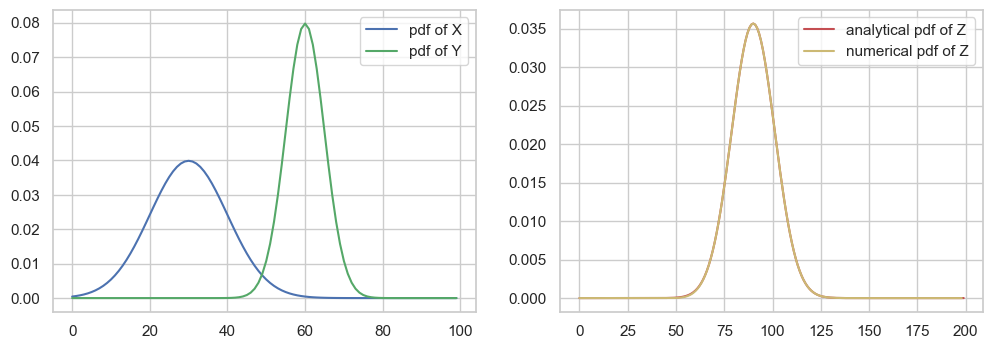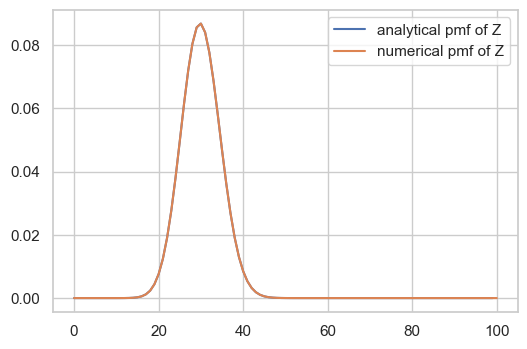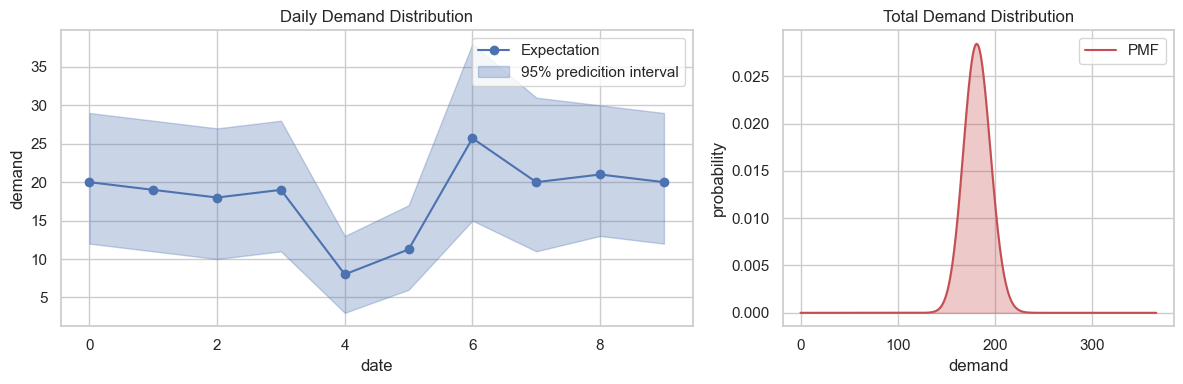## 附录

$\hat f(\omega) = \int_{-\infty}^{+\infty}f(t)\mathrm e^{-i\omega t}\mathrm dt$

$\hat g(\omega) = \int_{-\infty}^{+\infty}g(t)\mathrm e^{-i\omega t}\mathrm dt$

$h(t)$ 的傅里叶变换为

\begin{aligned} \hat h(\omega) &= \int_{-\infty}^{+\infty}h(t)\mathrm e^{-i\omega t}\mathrm dt\\ &= \int_{-\infty}^{+\infty}\left[\int_{-\infty}^{+\infty}f(\tau)g(t-\tau)\mathrm d\tau\right]\mathrm e^{-i\omega t}\mathrm dt\\ &= \int_{-\infty}^{+\infty}\int_{-\infty}^{+\infty}f(\tau)g(t-\tau)e^{-i\omega t}\mathrm dt\mathrm d\tau\end{aligned}

$s=t-\tau$，则 $\mathrm ds = \mathrm dt$

\begin{aligned} \hat h(\omega) & = \int_{-\infty}^{+\infty}\int_{-\infty}^{+\infty}f(\tau)g(s)e^{-i\omega (\tau+s)}\mathrm ds\mathrm d\tau\\ &= \int_{-\infty}^{+\infty}f(\tau)e^{-i\omega \tau}\mathrm d\tau\int_{-\infty}^{+\infty}g(s)e^{-i\omega s}\mathrm ds\\ &=\hat f(\omega)\cdot\hat g(\omega)\end{aligned}

## 参考文献

]]>
<p>我们在<a href="/2020/04/17/%E4%B8%80%E4%B8%AA%E6%9C%80%E5%A4%A7%E5%8C%96%E6%9D%A1%E4%BB%B6%E6%A6%82%E7%8E%87%E9%97%AE%E9%A2%98/" title="《一个最大化条件概率问题》">《一个最大化条件概率问题》</a>一文中提到，为了满足商品采购业务的需要，我们首先预测每一天的需求所服从的概率分布，然后计算若干天总需求所服从的概率分布。那么，如何将日需求的分布转化为总需求的分布呢？</p>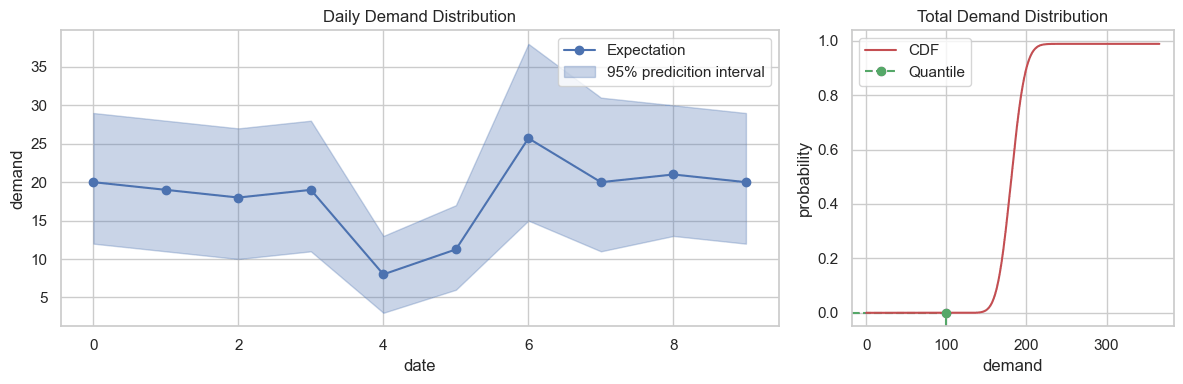## 问题

$X = (X_1, X_2, \cdots, X_n)\\Z = \sum_{i=1}^{n} X_i$

$\vec x^* = \max_{\vec x} P(X=\vec x|Z=z)$

## 求解

\begin{aligned} P(X=x|Z=z) &\propto f_n\left(z-\sum_{i=1}^{n-1}x_i\right)\cdot\prod_{i=1}^{n-1}f_i(x_i)\\ &\equiv g(x_1, x_2, \cdots, x_{n-1})\end{aligned}

$\eta(x) =\frac{f'(x)}{f(x)}$

\begin{aligned} \frac{\partial g}{\partial x_i} &= 0\\ &= -f_n'\left(z-\sum_{j=1}^{n-1}x_j\right)\cdot\prod_{j=1}^{n-1}f_j(x_j) + f_n\left(z-\sum_{j=1}^{n-1}x_j\right)\cdot\prod_{j=1, j\neq i}^{n-1}f_j(x_j)\cdot f_i'(x_i)\\ &= -\eta_n\left(z-\sum_{j=1}^{n-1}x_j\right)\cdot f_n\left(z-\sum_{j=1}^{n-1}x_j\right)\cdot\prod_{j=1}^{n-1}f_j(x_j) + f_n\left(z-\sum_{j=1}^{n-1}x_j\right)\cdot\prod_{j=1, j\neq i}^{n-1}f_j(x_j)\cdot f_i(x_i)\cdot\eta_i(x_i)\\ &= g\cdot(-\eta_n\left(z-\sum_{j=1}^{n-1}x_j\right) + \eta_i(x_i))\end{aligned}

$\eta_i(x_i) = \eta_n\left(z-\sum_{j=1}^{n-1}x_j\right)$

$\begin{cases} x_1 + x_2 + \cdots + x_n = z\\ \eta_1(x_1)=\eta_2(x_2)=\cdots=\eta_n(x_n)\end{cases}$

$\eta(x) = \frac{\mu-x}{\sigma^2}$

$\left[\begin{matrix} 1 & 1 & \cdots & 1 & 1\\ -1/\sigma_1^2 & 0 & \cdots & 0 & 1/\sigma_n^2\\ 0 & -1/\sigma_2^2 & \cdots & 0 & 1/\sigma_n^2\\ \vdots & \vdots & \ddots & \vdots & \vdots\\ 0 & 0 & \cdots & -1/\sigma_{n-1}^2 & 1/\sigma_n^2\end{matrix}\right]\left[ \begin{matrix} x_1\\x_2\\x_3\\\vdots\\x_n \end{matrix}\right]=\left[\begin{matrix} z\\ \mu_n/\sigma_n^2-\mu_1/\sigma_1^2\\ \mu_n/\sigma_n^2-\mu_2/\sigma_2^2\\ \vdots\\ \mu_n/\sigma_n^2-\mu_{n-1}/\sigma_{n-1}^2\\\end{matrix}\right]$

• 对于泊松分布，有

$\eta(x) = \ln\lambda - \psi(x+1)$

• 对于二项分布，有

$\eta(x) = \psi(n-x+1) - \psi(x+1) + \ln\frac{p}{1-p}$

• 对于负二项分布，有

$\eta(x) = \psi(x+r) - \psi(x+1) + \ln p$

$\psi(x) = \frac{\mathrm d}{\mathrm dx}\ln\left(\Gamma(x)\right)=\frac{\Gamma'(x)}{\Gamma(x)}$

• 对于泊松分布，有

$\eta'(x) = -\psi_1(x+1) <0$

• 对于二项分布，有

$\eta'(x) = -\psi_1(n-x+1) - \psi_1(x+1) <0$

• 对于负二项分布，有

$\eta'(x) = \psi_1(x+r) - \psi_1(x+1) <0\qquad if \quad r > 1$

$\psi_1(x) = \frac{\mathrm d^2}{\mathrm dx^2}\ln\left(\Gamma(x)\right)=\frac{\mathrm d}{\mathrm dx}\psi(x)$

\begin{aligned} \eta_{min} &= \max\left(\eta_1\left(z\right), \eta_2\left(z\right), \cdots, \eta_2\left(z\right)\right)\\ \eta_{max} &= \max\left(\eta_1\left(\frac zn\right), \eta_2\left(\frac zn\right), \cdots, \eta_2\left(\frac zn\right)\right)\end{aligned}

$\sum_{i=1}^{n}x_i^*=\sum_{i=1}^{n}\eta_i^{-1}(\eta^*) = z$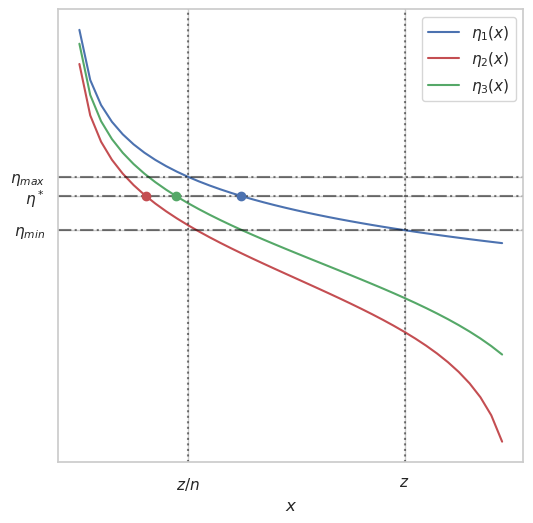## 代码

$\left[\begin{matrix} 1 & 1\\ -1 & 1/16\end{matrix}\right]\left[\begin{matrix} x_1\\x_2\end{matrix}\right]=\left[\begin{matrix} 5\\0\end{matrix}\right]$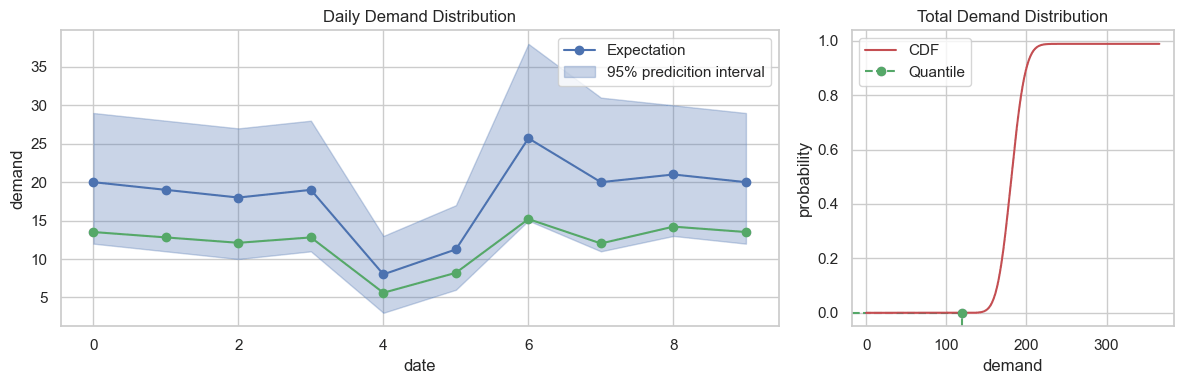## 附录

• 首先是正态分布，有

$f(x) = \frac{1}{\sigma\sqrt 2 \pi}\exp\left(-\frac{(x-\mu)^2}{2\sigma^2}\right)$

\begin{aligned} \eta(x) &= \frac{f'(x)}{f(x)}\\ &= \frac{\mathrm d}{\mathrm dx}\ln f(x)\\ &= \frac{\mathrm d}{\mathrm dx}\left(\ln\frac{1}{\sigma\sqrt 2 \pi}-\frac{(x-\mu)^2}{2\sigma^2}\right)\\ &= \frac{\mathrm d}{\mathrm dx}\left(-\frac{(x-\mu)^2}{2\sigma^2}\right)\\ &= \frac{\mu-x}{\sigma^2}\end{aligned}

• 其次是泊松分布，有

$f(x) = \frac{\mathrm e^{-\lambda}\lambda^x}{x!} = \frac{\mathrm e^{-\lambda}\lambda^x}{\Gamma(x+1)}$

\begin{aligned} \eta(x) &= \frac{f'(x)}{f(x)}\\ &= \frac{\mathrm d}{\mathrm dx}\ln f(x)\\ &= \frac{\mathrm d}{\mathrm dx}\left(-\lambda+x\ln\lambda-\ln\Gamma(x+1)\right)\\ &= \ln\lambda-\frac{\mathrm d}{\mathrm dx}\ln\Gamma(x+1)\\ &= \ln\lambda-\psi(x+1)\end{aligned}

• 接着是二项分布，有

\begin{aligned} f(x) & = \tbinom{n}{x}p^x(1-p)^{n-x}\\ &=\frac{n!}{x!(n-x)!}p^x(1-p)^{n-x}\\ &= \frac{\Gamma(n+1)}{\Gamma(x+1)\Gamma(n-x+1)}p^x(1-p)^{n-x}\end{aligned}

\begin{aligned} \eta(x) &= \frac{f'(x)}{f(x)}\\ &= \frac{\mathrm d}{\mathrm dx}\ln f(x)\\ &= \frac{\mathrm d}{\mathrm dx}\left(\ln\Gamma(n+1)-\ln\Gamma(x+1)-\ln\Gamma(n-x+1)+x\ln p+(n-x)\ln (1-p)\right)\\ &= \frac{\mathrm d}{\mathrm dx}\left(-\ln\Gamma(x+1)-\ln\Gamma(n-x+1)+x\ln p-x\ln (1-p)\right)\\ &= -\frac{\mathrm d}{\mathrm dx}\ln\Gamma(n-x+1)-\frac{\mathrm d}{\mathrm dx}\ln\Gamma(x+1) + \ln\frac{p}{1-p}\\ &= \psi(n-x+1) - \psi(x+1) + \ln\frac{p}{1-p}\end{aligned}

• 最后是负二项分布，有

\begin{aligned} f(x) &= \tbinom{x+r-1}{x}p^x(1-p)^r\\ &=\frac{(x+r-1)!}{x!(r-1)!}p^x(1-p)^r\\ &=\frac{\Gamma(x+r)}{\Gamma(x+1)\Gamma(r)}p^x(1-p)^r\\\end{aligned}

\begin{aligned} \eta(x) &= \frac{f'(x)}{f(x)}\\ &= \frac{\mathrm d}{\mathrm dx}\ln f(x)\\ &= \frac{\mathrm d}{\mathrm dx}\left(\ln\Gamma(x+r)-\ln\Gamma(x+1)-\ln\Gamma(r)+x\ln p+r\ln (1-p)\right)\\ &= \frac{\mathrm d}{\mathrm dx}\left(\ln\Gamma(x+r)-\ln\Gamma(x+1)+x\ln p\right)\\ &= \psi(x+r) - \psi(x+1) + \ln p\end{aligned}

## 参考文献

]]>
<h2 id="背景"><a class="markdownIt-Anchor" href="#背景"></a> 背景</h2> <p>我们在<a href="https://www.jianshu.com/p/dfac740faa34">《报童问题》</a>、<a href="/2019/11/26/%E6%8A%A5%E7%AB%A5%E9%97%AE%E9%A2%98%E7%9A%84%E7%AE%80%E5%8D%95%E8%A7%A3%E6%B3%95/" title="《报童问题的简单解法》">《报童问题的简单解法》</a>等文中介绍了一种通过考虑需求的不确定性来最大化销售利润的商品采购模型：首先预测需求所服从的概率分布，然后取能使得期望收益最大的分位数作为预估的需求，据此来决定采购量，对应的分位值定义为服务水平。</p> <p>在实际应用中，一次采购需要满足未来一段时间的总需求，具体是多长时间取决于商品的提前期和目标库转等因素。理论上我们可以直接预测这段时间的总需求所服从的概率分布。但站在甲方的角度，一段时间的总需求？还概率分布？没概念啊！你不告诉我每天的情况，直接丢一个最终结果给我，我怎么知道你靠不靠谱呢？</p>

]]>
<p>根据 <a href="https://google.github.io/styleguide/pyguide.html">Google Python Style Guide</a>，Python 中的参数名一般为 <code>lower_with_under</code> 风格。而根据 <a href="https://google.github.io/styleguide/javaguide.html">Google Java Style Guide</a>, Java 中的参数名一般为 <code>lowerCamelCase</code> 风格。那么问题来了，假如你写了一个 Python 算法服务供 Java 后端调用，设计接口的时候参数名应该采用什么命名风格呢？我工作中就遇到过这个问题。使用 Python 风格吧，后端同学用起来不方便；使用 Java 风格吧，作为一个强迫症我又很难受。有没有两全其美的办法呢？</p>

## 1. Quantile Loss

《分位数回归》一文中，我们证明了以最小化分位数损失作为训练目标，可以得到分位数预测模型。其实反过来看，分位数损失也可以作为概率分布预测的评估指标。

$Z_t$ 表示 $t$ 时刻的真实值，用 $\hat Z_t^\rho$ 表示概率分布预测给出的 $t$ 时刻的 $\rho$ 分位数，总共预测 $h$ 步，我们定义 Quantile Loss 为

$QL_\rho = 2\sum_{t=1}^{h}(\hat Z_t^\rho-Z_t)\left(\rho I_{\{\hat Z_t^\rho > Z_t\}} - (1-\rho)I_{\{\hat Z_t^\rho \leq Z_t\}}\right)$

$wQL_\rho = \frac{QL_\rho}{\sum\limits_{t=1}^h Z_t}$

$wQL_{0.5}=\frac{\sum_{t=1}^h|\hat Z_t^{0.5}-Z_t|}{ \sum_{t=1}^h Z_t} = wMAPE$

wMAPE 是在销量点预测中常用的评估指标，现在我们知道它可以看作分位数损失的一个特例，或者反过来说，分位数损失可以看作 wMAPE 的泛化。因此，选择分位数损失作为概率分布预测的评估指标还有一个额外的好处，就是可以把点预测和概率分布预测的评估统一起来。

## 2. Coverage

$C_\rho=\frac{1}{h}\sum_{t=1}^h I_{\{\hat Z_t^\rho \geq Z_t\}}$

\begin{aligned}\mathbb E I_{\{Z^\rho \geq Z \}} &= \int_{-\infty}^{+\infty} I_{\{ Z^\rho \geq z\}} f(z)\mathrm dz \\&= \int_{-\infty}^{Z^\rho} f(z)\mathrm dz \\&= F(Z^\rho) \\&= \rho\end{aligned}

## 3. MSIS (Mean Scaled Interval Score)

$MSIS = \frac{\frac{1}{h}\sum_{t=1}^h(\hat U_t-\hat L_t)+\frac{2}{\alpha}(\hat L_t-Z_t)I_{\{Z_t<\hat L_t\}} +\frac{2}{\alpha}(Z_t-\hat U_t)I_{\{Z_t>\hat U_t\}} }{\frac{1}{n-m}\sum_{t=m+1}^n|Z_t-Z_{t-m}|}$